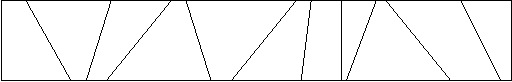# 计算几何——从入门到不会

## 计算几何相关注意事项

1. 注意题目让输入int型数据最好先直接输入int型数据，然后再赋给Point类中的double数据，因为直接输入double数据会比输入int慢很多（2s的题TLE换成输入int之后300ms直接AC）
2. 输出的时候尽量用printf，速度快且避免很多不必要的错误
3. 判断直线平行不要求斜率，直接用叉积，避免除号
4. 涉及比较大小的时候一定要用eps——dcmp()，精度问题十分重要

updated Sunday,11 June 2017

## POJ2318.Toys

Description

Calculate the number of toys that land in each bin of a partitioned toy box.
Mom and dad have a problem – their child John never puts his toys away when he is finished playing with them. They gave John a rectangular box to put his toys in, but John is rebellious and obeys his parents by simply throwing his toys into the box. All the toys get mixed up, and it is impossible for John to find his favorite toys.John’s parents came up with the following idea. They put cardboard partitions into the box. Even if John keeps throwing his toys into the box, at least toys that get thrown into different bins stay separated. The following diagram shows a top view of an example toy box.For this problem, you are asked to determine how many toys fall into each partition as John throws them into the toy box.

Input

The input file contains one or more problems. The first line of a problem consists of six integers, n m x1 y1 x2 y2. The number of cardboard partitions is n (0 < n <= 5000) and the number of toys is m (0 < m <= 5000). The coordinates of the upper-left corner and the lower-right corner of the box are (x1,y1) and (x2,y2), respectively. The following n lines contain two integers per line, Ui Li, indicating that the ends of the i-th cardboard partition is at the coordinates (Ui,y1) and (Li,y2). You may assume that the cardboard partitions do not intersect each other and that they are specified in sorted order from left to right. The next m lines contain two integers per line, Xj Yj specifying where the j-th toy has landed in the box. The order of the toy locations is random. You may assume that no toy will land exactly on a cardboard partition or outside the boundary of the box. The input is terminated by a line consisting of a single 0.

Output

The output for each problem will be one line for each separate bin in the toy box. For each bin, print its bin number, followed by a colon and one space, followed by the number of toys thrown into that bin. Bins are numbered from 0 (the leftmost bin) to n (the rightmost bin). Separate the output of different problems by a single blank line.

Sample Input

Sample Output

Hint

As the example illustrates, toys that fall on the boundary of the box are “in” the box.AC代码：

update  Saturday,8 July 2017

## Poj1269.Intersecting Lines

 Time Limit: 1000MS Memory Limit: 10000K Total Submissions: 15992 Accepted: 6923

### Description

We all know that a pair of distinct points on a plane defines a line and that a pair of lines on a plane will intersect in one of three ways: 1) no intersection because they are parallel, 2) intersect in a line because they are on top of one another (i.e. they are the same line), 3) intersect in a point. In this problem you will use your algebraic knowledge to create a program that determines how and where two lines intersect.
Your program will repeatedly read in four points that define two lines in the x-y plane and determine how and where the lines intersect. All numbers required by this problem will be reasonable, say between -1000 and 1000.

### Input

The first line contains an integer N between 1 and 10 describing how many pairs of lines are represented. The next N lines will each contain eight integers. These integers represent the coordinates of four points on the plane in the order x1y1x2y2x3y3x4y4. Thus each of these input lines represents two lines on the plane: the line through (x1,y1) and (x2,y2) and the line through (x3,y3) and (x4,y4). The point (x1,y1) is always distinct from (x2,y2). Likewise with (x3,y3) and (x4,y4).

### Output

There should be N+2 lines of output. The first line of output should read INTERSECTING LINES OUTPUT. There will then be one line of output for each pair of planar lines represented by a line of input, describing how the lines intersect: none, line, or point. If the intersection is a point then your program should output the x and y coordinates of the point, correct to two decimal places. The final line of output should read “END OF OUTPUT”.